##### Statistics For Dummies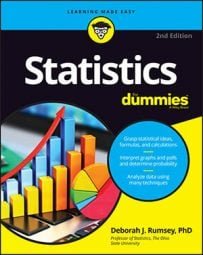When you set up a hypothesis test to determine the validity of a statistical claim, you need to define both a null hypothesis and an alternative hypothesis.

Typically in a hypothesis test, the claim being made is about a population parameter (one number that characterizes the entire population). Because parameters tend to be unknown quantities, everyone wants to make claims about what their values may be. For example, the claim that 25% (or 0.25) of all women have varicose veins is a claim about the proportion (that’s the parameter) of all women (that’s the population) who have varicose veins (that’s the variable — having or not having varicose veins).

Researchers often challenge claims about population parameters. You may hypothesize, for example, that the actual proportion of women who have varicose veins is lower than 0.25, based on your observations. Or you may hypothesize that due to the popularity of high heeled shoes, the proportion may be higher than 0.25. Or if you’re simply questioning whether the actual proportion is 0.25, your alternative hypothesis is: “No, it isn’t 0.25.”

## How to define a null hypothesis

Every hypothesis test contains a set of two opposing statements, or hypotheses, about a population parameter. The first hypothesis is called the null hypothesis, denoted H0. The null hypothesis always states that the population parameter is equal to the claimed value. For example, if the claim is that the average time to make a name-brand ready-mix pie is five minutes, the statistical shorthand notation for the null hypothesis in this case would be as follows: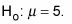(That is, the population mean is 5 minutes.)

## How to define an alternative hypothesis

Before actually conducting a hypothesis test, you have to put two possible hypotheses on the table — the null hypothesis is one of them. But, if the null hypothesis is rejected (that is, there was sufficient evidence against it), what’s your alternative going to be? Actually, three possibilities exist for the second (or alternative) hypothesis, denoted Ha. Here they are, along with their shorthand notations in the context of the pie example:

• The population parameter is not equal to the claimed value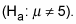• The population parameter is greater than the claimed value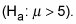• The population parameter is less than the claimed value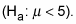Which alternative hypothesis you choose in setting up your hypothesis test depends on what you’re interested in concluding, should you have enough evidence to refute the null hypothesis (the claim). The alternative hypothesis should be decided upon before collecting or looking at any data, so as not to influence the results.

For example, if you want to test whether a company is correct in claiming its pie takes five minutes to make and it doesn’t matter whether the actual average time is more or less than that, you use the not-equal-to alternative. Your hypotheses for that test would be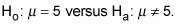If you only want to see whether the time turns out to be greater than what the company claims (that is, whether the company is falsely advertising its quick prep time), you use the greater-than alternative, and your two hypotheses are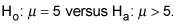Finally, say you work for the company marketing the pie, and you think the pie can be made in less than five minutes (and could be marketed by the company as such). The less-than alternative is the one you want, and your two hypotheses would be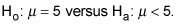How do you know which hypothesis to put in H0 and which one to put in Ha? Typically, the null hypothesis says that nothing new is happening; the previous result is the same now as it was before, or the groups have the same average (their difference is equal to zero). In general, you assume that people’s claims are true until proven otherwise. So the question becomes: Can you prove otherwise? In other words, can you show sufficient evidence to reject H0?